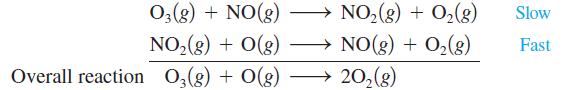# Problem: One mechanism for the destruction of ozone in the upper atmosphere isc. Ea for the uncatalyzed reactionO3 (g) + O (g) → 2O2 (g)is 14.0 kJ. Ea for the same reaction when catalyzed is 11.9 kJ. What is the ratio of the rate constant for the catalyzed reaction to that for the uncatalyzed reaction at 25°C? Assume that the frequency factor A is the same for each reaction.

###### FREE Expert Solution

Arrhenius Equation:

Ratio of rate constant for catalyzed to uncatalyzed:

$\frac{{\mathbf{k}}_{\mathbf{catalyzed}}}{{\mathbf{k}}_{\mathbf{uncatalyzed}}}\mathbf{=}\frac{\overline{)\mathbf{A}}{\mathbf{e}}^{\mathbf{-}\frac{{\mathbf{E}}_{\mathbf{a}\mathbf{,}\mathbf{cat}\mathbf{.}}}{\mathbf{RT}}}}{\overline{)\mathbf{A}}{\mathbf{e}}^{\mathbf{-}\frac{{\mathbf{E}}_{\mathbf{a}\mathbf{,}\mathbf{uncat}\mathbf{.}}}{\mathbf{RT}}}}$

T = 25°C + 273 = 298 K

80% (310 ratings)###### Problem Details

One mechanism for the destruction of ozone in the upper atmosphere isc. Ea for the uncatalyzed reaction

O3 (g) + O (g) → 2O2 (g)

is 14.0 kJ. Ea for the same reaction when catalyzed is 11.9 kJ. What is the ratio of the rate constant for the catalyzed reaction to that for the uncatalyzed reaction at 25°C? Assume that the frequency factor A is the same for each reaction.

Frequently Asked Questions

What scientific concept do you need to know in order to solve this problem?

Our tutors have indicated that to solve this problem you will need to apply the Arrhenius Equation concept. You can view video lessons to learn Arrhenius Equation. Or if you need more Arrhenius Equation practice, you can also practice Arrhenius Equation practice problems.

What professor is this problem relevant for?

Based on our data, we think this problem is relevant for Professor Keister's class at UB.

What textbook is this problem found in?

Our data indicates that this problem or a close variation was asked in Chemistry: An Atoms First Approach - Zumdahl Atoms 1st 2nd Edition. You can also practice Chemistry: An Atoms First Approach - Zumdahl Atoms 1st 2nd Edition practice problems.